## Fixed one end, pinned the other, beam calculator

This tool calculates the static response of beams, with one end fixed, and the other simply supported, under various loading scenarios. The tool calculates and plots diagrams for these quantities:

• reactions
• bending moments
• transverse shear forces
• deflections
• slopes

Please take in mind that the assumptions of Euler-Bernoulli beam theory are adopted, the material is elastic and the cross section is constant over the entire beam span (prismatic beam).

Units:
 1 2 3 4 Structure L = mcmmmydftin Optional properties, required only for deflection/slope results: E = PakPaMPaGPapsiksiMpsi I = m^4cm^4mm^4ft^4in^4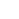Calculate the moment of inertia of various beam cross-sections, using our dedicated calculators.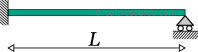ADVERTISEMENT
 1 2 3 4 Results: Reactions: RA = kNNkgtlbfkip RB = kNNkgtlbfkip MA = kNmNmkg.mt.mlbf.ftlbf.inkip.ftkip.in Bending Moment: Mu = kNmNmkg.mt.mlbf.ftlbf.inkip.ftkip.in xm = mcmmmydftin Transverse Shear Force: Vu = kNNkgtlbfkip xv = mcmmmydftin Deflection: du = mcmmmydftin xd = mcmmmydftin Slopes: θA = degradmrad θB = degradmrad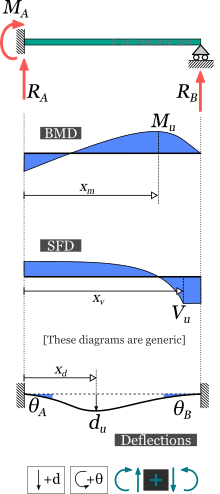1 2 3 4 Request results at a specific point: x = mcmmmydftin M(x) = kNmNmkg.mt.mlbf.ftlbf.inkip.ftkip.in V(x) = kNNkgtlbfkip d(x) = mcmmmydftin θ(x) = degradmrad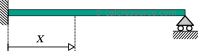## Theoretical background

### Introduction

The fixed at one end beam and simply supported at the other (will be called fixed-pinned for simplicity), is a simple structure that features only two supports: a fixed support and a pinned support (also called hinge). Fixed supports inhibit all movement, including vertical or horizontal displacements as well as rotations. On the other hand, pinned supports (also called hinged), inhibit displacements but permit rotations to occur freely. As a result, a typical deflected geometry, of the fixed at one end beam, appears with zero slope, at the fixed support, and non-zero slope, at the hinged end. This is illustrated in the following figure.The fixed-pinned beam features one fixed support and a pinned support

The fixed-pinned beam features more supports than required to be statically effective. Indeed the pinned support could be removed entirely, turning the structure to a cantilever beam, which is still an effective load bearing structure. In other words, the fixed-pinned beam offers redundancy in terms of supports. To the contrary, a structure without redundancy, would become a mechanism, if any of its supports were removed, or an internal hinge was introduced. A mechanism can move without restriction in one or more directions, an unwanted situation of a load bearing structure. For this reason the structures that offer no redundancy, are called critical or determinant structures. If a local failure occurs to these structures, they would collapse. To the opposite, a structure that features more supports than required to restrict its free movements, is called redundant or indeterminate structure. Redundant structures can tolerate one or more local failures before they become mechanisms. The fixed-pinned beam is an indeterminate (or redundant) structure.

#### Assumptions

Typically, when performing a static analysis of a load bearing structure, the internal forces and moments (commonly referred as resultants), as well as the deflections must be calculated. When the structure is 2-dimensional, and the imposed loads are exercised in the same 2D plane, there are just three resultant actions of interest:

1. the axial forces ,
2. the transverse shear forces , and the
3. bending moments .

For a beam, fixed at one end and pinned at the other, which is loaded by transverse loads only (so that their direction is perpendicular to the beam longitudinal axis), the axial force is always zero, provided the deflections remain small. As a result, it is common for the axial forces to be neglected.

The response resultants and deflections presented in this page are calculated taking into account the following assumptions:

• The material is homogeneous and isotropic (in other words its characteristics are the same in ever point and towards any direction).
• The material behavior is linear and elastic.
• The loads are imposed in a static manner (they do not change rapidly with time).
• The cross section is the same throughout the beam length (i.e. the beam is prismatic).
• The deflections remain small.
• Every cross-section that initially is plane and also normal to the longitudinal axis, remains plane and and normal to the deflected axis too. This is the case when the cross-section height is quite smaller than the beam length (10 times or more) and also the cross-section is not multi layered (not a sandwich type section).

The last two assumptions satisfy the kinematic requirements for the Euler-Bernoulli beam theory and are adopted here too.

#### Sign convention

A sign convention is more than convenient for the calculation of the internal forces and moments, , and their physical interpretation, at any section cut throughout the beam. The following set of rules is adopted in this page:

1. The axial force, , is considered positive when it causes tension to the part.
2. The shear force, , is positive when it causes a clock-wise rotation of the part.
3. The bending moment, , is positive when it causes tension to the lower fiber of the beam and compression to the top fiber.

These rules, though not mandatory, are rather universal. A different set of rules, if followed consistently would also produce the same physical results.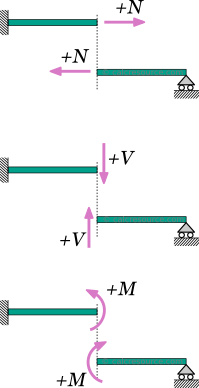Positive sign convention for internal axial force, N, shear force, V, and bending moment, M

#### Symbols

• : the material modulus of elasticity (Young's modulus)
• : the moment of inertia of the cross-section around the elastic neutral axis of bending
• : the total beam span
• : support force reaction
• : deflection
• : bending moment and support moment
• : transverse shear force
• : slope
• : total force of a distributed load

### Fixed at one end beam with uniform distributed load

In this case, the load is distributed uniformly, over the entire beam span, having constant magnitude and direction. Its dimensions are force per length. The total amount of force applied to the beam is , where , is the span length. In some cases the total force may be given rather than the force per length .

The following table presents the formulas describing the static response of a fixed at one end beam and pinned at the other, under a uniform distributed load .

### Fixed at one end beam with point force in the middle

In this case, the force is concentrated in a single point, located in the middle of the beam. In practical terms however, the force could be exercised over a limited length. In the close vicinity of the force application, stress concentrations are expected. As a result, the response predicted by the classical beam theory is maybe inaccurate. This is only a local phenomenon however. As we move away from the force location, the results become valid, by virtue of the Saint-Venant principle, provided the area where the load is imposed, remains substantially smaller than the total beam length.

The following table presents the formulas describing the static response of a fixed at one end beam and hinged at the other, under a concentrated point force , imposed in the middle.

### Fixed at one end beam with point force at a random position

In this case, the force is concentrated in a single point, anywhere across the beam span. If that is in the middle of the span (i.e. ), then the formulas presented in these section are perfectly valid, though for this particular case, the formulas presented in the previous section, are quite more convenient. In practical terms, the imposed force could be exercised on a small area, rather than an infinitesimal point. In order to consider the force as concentrated, the dimensions of the loading area should be considerably smaller than the total beam length. In the close vicinity of the force, stress concentrations are expected and as result the response predicted by the classical beam theory is maybe lacking. Nevertheless, this is only a local phenomenon. As we move away from the force, the discrepancy of the results becomes insignificant.

The following table presents the formulas describing the static response of a fixed at one end beam and pinned at the other, under a concentrated point force , imposed at a random distance from the left end.

### Fixed at one end beam with point moment

In this case, a moment is imposed in a single point of the beam, anywhere across the beam span. In practical terms, it could be a force couple, or a member in torsion, connected out of plane and perpendicular to the beam, as illustrated in the following figure.

Practically, the moment cannot be exercised on an ideally infinitesimal point. Instead, a small length of the beam, would be subject to the imposed moment action. In the close vicinity the loading area, the predicted results through the classical beam theory are expected to be inaccurate (due to stress concentrations and other localized effects). However, as we move away, the predicted results become perfectly valid, as stated by the Saint-Venant principle, provided the loading area remains substantially smaller than the total beam length.

The following table presents the formulas describing the static response of a fixed at one end beam and pinned at the other, under a concentrated point moment , imposed at a distance from the left end.

### Fixed at one end beam with trapezoidal load

In this case, the load is distributed over the entire beam length, with magnitude varying linearly, starting from at the left end, up to at the right end. The dimensions of and are force per length. The total amount of force applied to the beam is: , where the beam length.The values of and can be freely assigned. It is not mandatory for the former to be smaller than the latter. They may take even negative values (one or both of them).If , the formulas in the following table correspond to a triangular distributed load, with increasing magnitude (peak at the hinged end)If , the formulas in the following table correspond to a triangular distributed load, with decreasing magnitude (peak at the fixed end).

The following table presents the formulas describing the static response of a fixed at one end beam and pinned at the other, under a varying distributed load, of trapezoidal shape.

### Fixed at one end beam with slab-type trapezoidal load distribution

This is a load distribution common for beams in the perimeter of a slab. The shape of the distributed load is trapezoidal, as illustrated in the following figure. The peak load magnitude is , occurring at the interior of the beam, while at the two sides the load is linearly varying, decreasing to zero at the two end points. The dimensions of are force per length. The total amount of force applied to the beam is: , where the beam length and , the left and right side lengths, respectively, where the load distribution is linearly varying.

The following table presents the formulas describing the static response of a fixed at one end beam and hinged at the other, under a trapezoidal load shape, as depicted in the schematic.

### Fixed at one end beam with partially distributed uniform load

In this case the load is distributed uniformly to only a part of the beam, with a constant magnitude . The rest of the beam remains free of any load. The dimensions of are force per length. The total amount of force applied to the beam therefore is: , where is the beam length and , are the left and right side lengths, respectively, where no load is imposed.

The following table presents the formulas describing the static response of the fixed at one end beam and simply supported at the other, under a partially distributed uniform load.

### Fixed at one end beam with partially distributed trapezoidal load

In this case, the load is distributed to only a part of the beam, while the remaining length remains unloaded. The load distribution is varying linearly in magnitude from , towards the left side, up to , towards the right side. The dimensions of and are force per length. The total amount of force applied to the beam is therefore: , where is the beam length and , are the lengths at the left and right side of the beam, respectively, where no load is imposed.The values of and can be freely assigned. It is not mandatory for the former to be smaller than the latter. They may take even negative values (one or both of them).This is the most generic case. The formulas for partially distributed uniform and triangular loads can be derived by appropriately setting the values of and . Furthermore, the respective cases for fully loaded span, can be derived by setting and to zero.

The following table presents the formulas describing the static response of the fixed at one end beam and pinned at the other, under a partially distributed trapezoidal load.

### Related articles

Simply supported beam calculator
Cantilever beam calculator
Fixed beam calculator
All Moment of Inertia tools
Moments of Inertia Table
All calculators on Statics